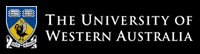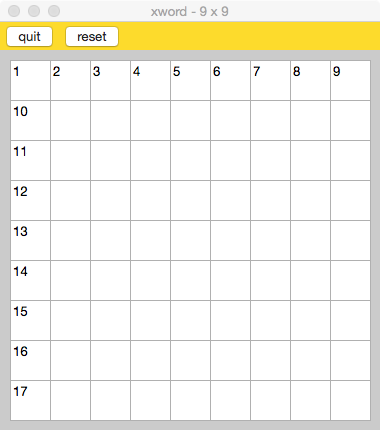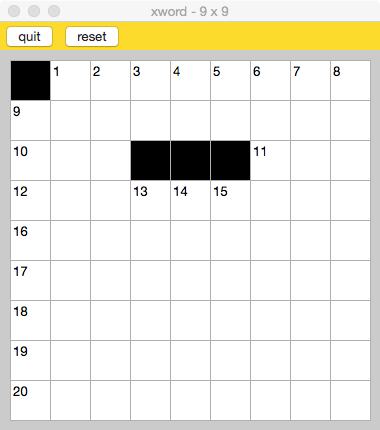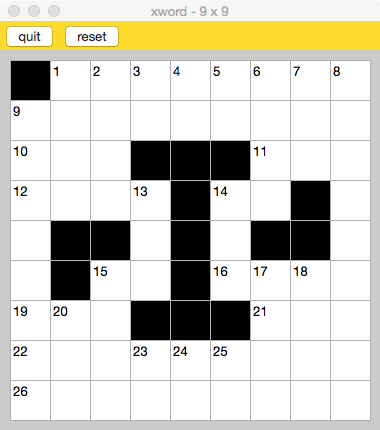CITS2002 Systems Programming

# Department of Computer Science and Software Engineering

## CITS2002 Systems Programming

### Labsheet 3 - for the week commencing 17th August 2020

The functions you write should be called from the main() function of your program. Your functions should receive all required values as parameters to the function and (if needed) return a result to the calling function. For example, in the code below, the process() function receives a string argument (the first command-line argument to your program) and returns an integer value of 0. The calling function (the main() function) stores the return result of the process() function in a variable called answer, and then prints this value to the screen via the printf() function.

 ```#include #include int process_argument(char s[]) { // DO SOMETHING WITH PARAMETER s ... return 0; } int main(int argc, char *argv[]) { // ENSURE THAT PROGRAM HAS CORRECT NUMBER OF ARGUMENTS if (argc < 2) { fprintf(stderr, "Usage: %s argument\n", argv); exit(EXIT_FAILURE); } else { // CALL THE PROCESS FUNCTION AND COLLECT THE RESULT int answer = process_argument(argv); // PRINT THE RESULT printf("The answer is %d\n", answer); // TERMINATE PROGRAM, INDICATING SUCCESS exit(EXIT_SUCCESS); } return 0; } ```

Note that the return datatype of the process_argument() function could be changed to any datatype we require (e.g. to a bool), depending on the task the process_argument() function is performing. Of course, the datatype of the answer variable and the printf() specifier used to print the value would also need to change.

1. Write a function named my_strlen() that calculates and returns the length of a string. Your function should take one argument, a character array that represents the string, and return an integer - the length of the string. The calling function (the main() function) should print the integer returned by your my_strlen() function.

Test your function with some string constants and by passing to it some command-line arguments.

2. A computer password is often consider "safe" (i.e. hard to guess) if it contains a mixture of uppercase and lowercase characters and digits. Write a function named isSafe() to determine if a potential password (a string) has at least two uppercase characters, two lowercase characters, and two digits. Your function should take a single character array as its argument and return a Boolean value to indicate whether the password is considered safe or not.

See the online documentation (man pages) for help with the isalpha(), islower(), and isupper() functions. Include the appropriate header file <ctype.h> into your program.

3. Write a function named my_strcmp() to determine if one string is (lexicographically, or alphabetically) less than, equal to, or greater than another string. Your function should accept the two character arrays as its arguments, and return either: -1 if the first string is less than the second string, 0 if the two strings are equal, or 1 if the first string is greater than the second string.

Call your function from the main() function with the code: my_strcmp(argv, argv).

4. Each call to the standard C99 function rand() returns a different random integer. Running the same program multiple times results in exactly the same sequence of random integers. While this is generally unexpected ("hey, they are not random"!), it is very helpful for debugging programs without the randomness.

We can provide each execution with a more random sequence of random numbers by seeding the random number generator with the C statement srand( time(NULL) );

• Write a simple program to fill an array of 10 integers with random numbers from rand(). Run the program several times, printing the contents of the array.
• Now, use srand() to seed the generation of random numbers. Run the program several times, printing the contents of the array.
• Extend your program by passing the initialised array to another function which finds and prints the largest value in the array.
• Finally, extend the program's function to place the array's largest value into the array's first element (index position 0), "pushing" all other values down in the array (0→1, 1→2, 2→3, and so on).

5. 🌶 Write a function named replace() that replaces all instances of one string for another string in a third string. For example:

prompt> ./replace red blue aredrobin abluerobin prompt> ./replace cat bison catocathartic bisonobisonhartic

A reasonable prototype for the function is:

` void replace( char oldword[], char newword[], char whole_sentence[] );`

Ensure you have terminated your string correctly.

6. 🌶 🌶 Consider a standard (square) crossword puzzle grid. Each square of the grid is black, or white (empty), or holds the number of a clue. A word (with at least two characters, and having a matching clue) commences from a numbered square, either across or down. Two clues can commence from the same numbered square, and can proceed both across and down.

To complete this exercise you'll need the Tcl/Tk graphical toolkit installed on your computer.

You will only need to modify/extend the file xword.c

You can run the program by clicking on a grid square, which will toggle the square between being black or white. Within the click() function you should also re-number the squares by setting those squares requiring a clue number to a positive value. The grid will then be automatically redrawn. As execution continues, the mechanism required to correctly re-number the squares should become apparent. For example:7. 🌶 🌶 🌶 If you had to write some code that iterated through all the possibilities for three variables in the range 0 to 10, you would probably write code similar to:

 ```for(int a = 0; a < 10; ++a) { for(int b = 0; b < 10; ++b) { for(int c = 0; c < 10; ++c) { ; // LOOP-BODY USING a, b, AND c } } } ```

But what if you had to extend this to 4, 5, or even 10+ "nested" loops? Instead of further nesting more loops, it is possible to write a function that acts as a "superloop", performing the equivalent of n nested loops with just one loop. Write a function to do this, taking an argument n that indicates the number of nested loops your function should perform. To do this, you will need to keep a 1-dimensional array of n values that maintain the state of each loop during the execution of your function.

# The University of Western Australia

## University information

CRICOS Code: 00126G